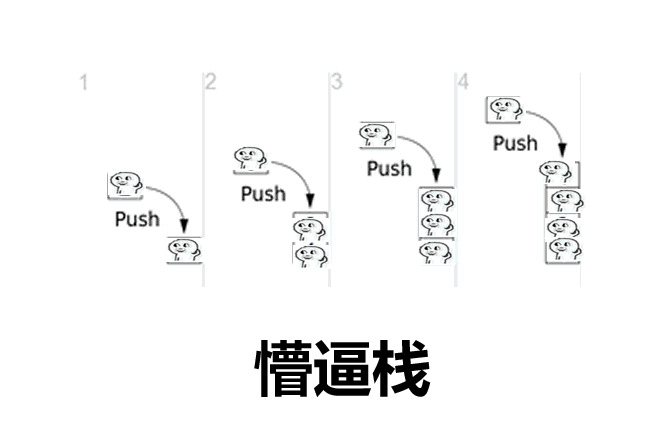• # Bio

祝所有人 AK IOI、AK CSP-J、AK CSP-S、AK NOIP、AK NOI、AK APIO、AK CTSC、AK ROI、AK WC、AK NEVER、AK GONNA、AK GIVE、AK YOU、AK UP自己做的小 Logo ↑ ↑ ↑

今年 NOI 题解 & 题目：点（我不建议你点，会后悔）

${\color{Purple} \mathfrak{Welcome!}}$

${\color{Orange} \mathbb{Main\space\ Content} }$

$\small\texttt{\color{#FA4129}本}\huge\texttt{\color{#FE9019}人}_{\small\texttt{\color{#FFE304}的}^{\large\texttt{\color{#FFEC01}萌\color{#FFF900}新}\small\texttt{\color{#FCFB03}Q\color{#F8FB07}w\color{#F1FB0B}Q}}}^{\large\texttt{\color{#FFB511}是}{\small\texttt{\color{#FFDC07}刚\color{#FFEF00}学}\large\texttt{\color{#FFF600}CSP\color{#FFFA00}-S}}}\huge\texttt{\color{#E6F911}但\color{#92E82F}是}^{\large\texttt{\color{#39D54B}即}{\small\texttt{\color{#03C767}使}}}_{\normalsize\text{\color{#07C964}是\color{#00C789}这\color{#00C7A5}样}}\texttt{\color{#00CBC6}我\color{#00D0EB}也}^{\small\texttt{\color{#00D0F2}要}\normalsize\texttt{\color{#00D0F6}用}\texttt{\color{#03BEF4}stu}_{\texttt{\color{#04AAEF}dent}\large\texttt{\color{#078DE4}的}}}_{\scriptsize\texttt{\color{#01CDF6}声\color{#03C2F5}音\color{#04B4F2}大\color{#04A7EE}声\color{#0791E6}喊\color{#0A7BDD}出}}\mathcal{\color{#125BCD}I\space\color{#3D2AB5}AK\space\color{#A011AD}IOI!}$

每日膜拜：

%%%Leo2011

%%%qiqi2011

%%%Allen123

日记：

• 2023

• Oct. 10. ：DP难死了....还要自己推到，DFS DP 这类的都是抽象的玩意（悲
• Sep. 30th. ：Dijkstra 我什么时候才能弄懂啊啊啊！！
• Oct. 2nd. ：CSP-J初赛没过，****的S过了，现在S组第一题都是DP服了
• Nov. 26th. ：班里有早恋的怎么办 kky/qz QwQ
• Nov. 29th. ：数学竞赛了，题真TAM难！

$\frac{1^2+2^2}{1\times 2}+\frac{2^2+3^2}{2\times 3} +......+\frac{2027^2+2028^2}{2027\times 2028}$

• Nov. 30th. ：测吉日/时 可以帮助我这一天的快乐，算命是多么“好”的工具啊
• Dev. 1st. ：默默喜欢上了 Beyond
• Dev. 3rd. : 我在苦思冥想：

$\begin{array}{c} \sqrt[]{64}=8，因为 8\times 8=64 \\ i = \sqrt[]{-1} \\ e^{i\pi } = \cos \pi +i\sin \pi =-1\\ \end{array} e=1+\sum_{k=1}^{\infty }\frac{1}{k!} =1+\frac{1}{1!} +\frac{1}{2!} +......+\frac{1}{\infty !}\\ 所以 i=?$

$dp[i]=\max(dp[i-rp]+dp[i-j] )+RP·10^\infty\\ \mathbf{RP++}$

失败是成功之母，不要有任何的欲望心，顺其自然，日积月累，不要把自己的事情放大化，要静下心来，认真的分析

如果你不知道解题思路，那么你可以换一种思考方式，不需要在这个问题上纠结。比赛前若出现不会的题目，一定要积极的问老师，不要自己憋着，硬说自己要努力悟出来，你如果问了老师，老师会有更好的方式来改变你的思想、思路、方式

俗话说得好，有志者，事竟成，一个伟大的人都有一个伟大的思想，任何伟大的思想都有一个微不足道的开始；就在我们眼前的开始，对待你的是种种考验，你要真心对待你的所有考验，因为这些都是训练你的精华

金玉其外败絮其中，没必要追求## 人生哲理（bushi）

这确实是一个很难的问题，这个问题我们需要从根源进入，那么，为什么这个问题需要呢？是因为这个问题被提出来了，那么它就被提出来了，为什么呢？是之所以是个问题是因为它需要被解决，如果不解决他就不是个问题，我们需要解决那么就要解决，Because so（因为所以）是经典名言，黑格尔曾经说过：“四十二号混凝土是可以拌意大利面的，因为这个三角函数是会影响到整个核污染的大海和充电器，所以头皮屑是碳酸钙”

个人认为这是解决问题的根本问题

详细解释如下：

根据Never Gonna Give You Up定理，可以算出 $a^2+b^2=c^{114514}$$6 = 1-14+5+14$，然后根据 $O_{114514}$ 臭氧的组成：

$O_{114514}=Never Gonna Give You Up+sb +1145$

然后再根据法律第1919810条“不结婚禁止离婚”的条约可以总结出：

$\left( \sum_{k=1}^n a_k b_k \right)^{\!\!2}\leq \left( \sum_{k=1}^n a_k^2 \right) \left( \sum_{k=1}^n b_k^2 \right)\\\begin{array}{c} S= \binom{N}{n},A_{k}=\binom{M}{k}\cdot \binom{N-M}{n-k} \\ P\left ( A_{k}\right ) = \frac{\binom{M}{k}\cdot \binom{N-M}{n-k}}{\binom{N}{n}} \end{array}\\\sin \alpha + \sin \beta =2 \sin \frac{\alpha + \beta}{2}\cos \frac{\alpha - \beta}{2} \\\begin{array}{l} \text{对于方程形如：}x^{3}-1=0 \\ \text{设}\text{:}\omega =\frac{-1+\sqrt{3}i}{2} \\ x_{1}=1,x_{2}= \omega =\frac{-1+\sqrt{3}i}{2} \\ x_{3}= \omega ^{2}=\frac{-1-\sqrt{3}i}{2} \end{array} \\所以 1+1=nevergonna, O_{114514}=give you up$

不仅如此，我们根据因为所以科学道理（Because so）可以再次推理：

皇帝碳酸钙躺在叶酸里英年早逝，享年3000岁

为什么呢？因为所以科学道理

而且还有圆的面积公式：Would like doing sb sth

${\color{green}{ \mathcal{OTHER\space CONTENT} }}$

码风（自己可以猜猜题目）：

// 最小生成树模板  洛谷P3366
#include <bits/stdc++.h>

using namespace std;

const int N = 2e5 + 10;
int n, m, ans, cnt;
int fa[N];
struct node {
int x, y, len;
}e[N];
bool cmp(node a, node b) {
return a.len < b.len;
}

int dfs(int x) {
return fa[x] == x ? x : fa[x] = dfs(fa[x]);
}

void init() {
for (int i = 1; i <= m; i++) {
fa[i] = i;
}
}

void kruskal() {
for (int i = 1; i <= m; i++) {
int fx = dfs(e[i].x);
int fy = dfs(e[i].y);
if (fx != fy) {
fa[fx] = fy;
ans += e[i].len;
cnt++;
}
}
}

int main() {
cin >> n >> m;
init();
for (int i = 1; i <= m; i++) {
scanf("%d%d%d", &e[i].x, &e[i].y, &e[i].len);
}
sort(e+1, e+m+1, cmp);
kruskal();
if (cnt == n-1) {
cout << ans;
} else {
cout << "orz";
}
return 0;
}

#include <bits/stdc++.h>

using namespace std;

int dp, w, c, cnt = 0;

int main() {
int n, m;
cin >> n >> m;
for (int i = 0; i < n; i++) {
int x, y, z;
cin >> x >> y >> z;
int s = 1;
while (s < z) {
w[cnt] = 666666;
c[cnt++] = s * y;
z -= s;
s *= 2;
}
if (z != 0) {
w[cnt] = z * x;
c[cnt++] = z * y;
}

}
for (int i = 0; i < cnt; i++) {
for (int j = m; j >= w[i]; j--) {
dp[j] = max(dp[j], dp[j-w[i]] + c[i]);
}
}
cout << dp[m];
return 0;
}

#include <bits/stdc++.h>

using namespace std;

int n, a;
queue<int> q;
void bfs(int x) {
q.push(x);
while (q.size()) {
int t = q.front();
q.pop();
cout << t << " ";
if (a[t]) {
q.push(a[t][?]);
}
if (a[t]) {
q.push(a[t][?]);
}
}
}

int main() {
cin >> n;
for (int i = 1; i <= n; i++) {
int ax, b, c;
cin >> ax >> b >> c;
a[ax] = b;
a[ax] = c;
}
bfs(1);
return 0;
}unsigned long long rp = 0;
rp--;
cout << rp;


# 论：一个人是怎么把 dfs 写出来的

void dfs(int u) {  // 简简单单
vis[u] = 1;
for (int i = head[u]; i; i = e[i].x) {
if (!vis[e[i].t]) {
dfs(v);
}
}
}

https://www.luogu.com.cn/problem/P6838


3

2
Special Judge
2
2004
1
2007
1

1
NOIp 提高组
1

1

1

1

1

1

1

1

1

1

1

1

1

1